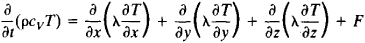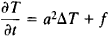# Heat Equation

(redirected from Heat Diffusion)

## heat equation

[′hēt i‚kwā·zhən]
(thermodynamics)
A parabolic second-order differential equation for the temperature of a substance in a region where no heat source exists: ∂ t /∂τ = (kc)(∂2 t /∂ x 2+ ∂2 t /∂ y 2+ ∂ t 2/∂ z 2), where x, y, and z are space coordinates, τ is the time, t (x,y,z, τ) is the temperature, k is the thermal conductivity of the body, ρ is its density, and c is its specific heat; this equation is fundamental to the study of heat flow in bodies. Also known as Fourier heat equation; heat flow equation.
McGraw-Hill Dictionary of Scientific & Technical Terms, 6E, Copyright © 2003 by The McGraw-Hill Companies, Inc.
The following article is from The Great Soviet Encyclopedia (1979). It might be outdated or ideologically biased.

## Heat Equation

a parabolic partial differential equation that describes the process of propagation of heat in a continuous medium, such as a gas, liquid, or solid. It is the basic equation in the mathematical theory of thermal conductivity.

The heat equation expresses the heat balance for a small element of volume of the medium; heat gains from sources and heat losses through the surface of the element are taken into account for heat transport by conduction. The equation has the following form for an isotropic nonhomogeneous medium:Here, ρ is the density of the medium; cv is the specific heat of the medium at constant volume; t is time; x, y, and z are space coordinates; T = T(x, y, z, t) is the temperature, which is calculated by means of the heat equation; λ is the coefficient of thermal conductivity; and F = F(x, y, z, t) is the specified density of the heat sources. The magnitudes of ρ cv, and λ depend on the coordinates and, generally speaking, on the temperature. For an anisotropic medium, the heat equation contains in place of λ the thermal conductivity tensor λik, where i, k = 1,2,3.

In the case of an istropic homogeneous medium, the heat equation assumes the formwhere Δ is the Laplace operator, a2 = λ/(ρcv) is the coefficient of thermal diffusivity, and f = F/(ρcv). In a stationary state, where the temperature does not vary with time, the heat equation becomes the Poisson equation or, when there are no heat sources, Laplace’s equation ΔT = 0. The basic problems for the heat equation are the Cauchy problem and the mixed boundary value problem (seeBOUNDARY VALUE PROBLEMS).

The heat equation was first studied by J. Fourier in 1822 and S. Poisson in 1835. Important results in the study of the heat equation were obtained by I. G. Petrovskii, A. N. Tikhonov, and S. L. Sobolev.

### REFERENCES

Carslaw, W. S. Teoriia teploprovodnosti. Moscow-Leningrad, 1947.
Vladimirov, V. S. Uravneniia matematicheskoi fiziki. Moscow, 1967.
Tikhonov, A. N., and A. A. Samarskii. Uravneniia matematicheskoi fiziki, 3rd ed. Moscow, 1966.

D. N. ZUBAREV

References in periodicals archive ?
Perona and Malik proposed nonlinear heat diffusion algorithm for noise reduction in 1990 , but the requirement of user-defined parameters was the main drawback of the method.
In this paper, a noninvasive method is proposed to measure blood flow velocity of a finger via heat diffusion between a finger and a ring.
Recently, hexagonal Boron Nitride (h-BN) bottom substrate can be a great heat drained material for disordered graphene so as to extremely reduce the carrier-phonon scattering via Wiedemann-Franz law heat diffusion, which is [delta] = 2 [15-17].
where [alpha]([m.sup.2]/s) and D([m.sup.2]/s) are heat diffusion coefficient and mass diffusion coefficient respectively.
Hristov, "Transient heat diffusion with a non-singular fading memory: from the cattaneo constitutive equation with Jeffrey's kernel to the caputo-fabrizio time-fractional derivative," Thermal Science, vol.
TEFAL's innovative 4-layer ceramic coated pot has a unique diamond-shaped pattern that contributes to even heat diffusion and better cooking, resulting in tasty and fluffy rice.
However, predefined heat source with Gaussian distribution and (2D) asymmetric model were examples of simplifications adopted by some authors in order to solve the numerical heat diffusion equation.
The light delivered in short pulse, the local temperature will increase rapidly because the heat diffusion was minimized [15, 13, 16].
The editors have organized the lectures that make up the main body of the text in sections devoted to the scholar who presented them, beginning with Gerhard HuiskenAEs lecture on heat diffusion in geometry and concluding with Brian WhiteAEs four lecture series introducing minimal surface theory.
This is achieved by heating tissue through heat diffusion, leading to a nice tightening effect on the skin.
In bio-electromagnetics, we are dealing with several issues including (1) Static-microwave-TeraHertz (0.1 to 10THz), (2) medical, diagnosis, cell phones, hyperthermia, exposure, imaging, thermotherapy, (3) SAR (Specific Absorption Rate), thermotherapy, bio-effects of magnetic field, eddy current, and (4) heat diffusion.

Site: Follow: Share:
Open / Close